## Frequency Response of Common Mode Gain of Differential Amplifier:

Low values of common-mode gain are desirable so that the circuit can reject undesirable signals that are applied equally to both inputs. The common-mode (CM) gain becomes important because undesired common-mode signals may have high-frequency components.

The important aspects of the Frequency Response of Common Mode Gain of Differential Amplifier can be calculated with some approximations. Consider the time constant=RTCT, where RT and CT are the equivalent output resistance and capacitance of the tail current source and RT is usually greater than or equal to output resistance of a transistor.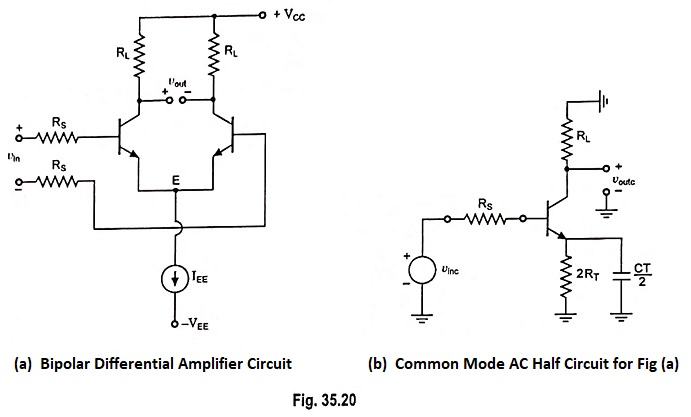Let us assume that the resistance RT is of the order 1 MΩ. The capacitor CT includes capacitance of current source transistor typically CT = 1 pF or less.

Thus time constant = RTCT = 1 MΩ x 1 pF = 1 μs and the break frequency corresponding to this time constant is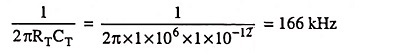Below this frequency the impedance ZT is dominated by RT, and above this frequency CT dominates. Thus as the operating frequency is increased, the impedance ZT will exhibit frequency variation before the rest of the circuit.

Assume that CT is the only significant capacitance. Since the impedance ZT is high, almost all of vin c appears across ZT if source resistance Rs is small. Thus common-mode gain can be approximated as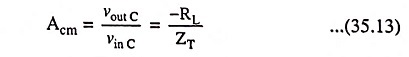where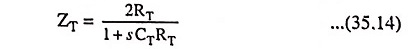From Eqs. (35.13) and (35.14) we have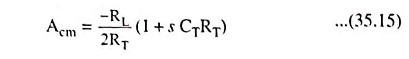Above Eq. (35.15) reveals that the common-mode gain expression contains a zero, which causes the common-mode gain to rise at 6 dB/octave above a frequency ω = 1/RTCT. This behavior is undesirable because the common-mode gain should ideally be as small as possible.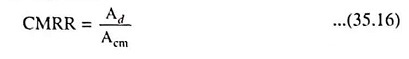The CMRR is plotted as a function of frequency in Fig. 35.21(c) by simply taking the magnitude of the ratio of the differential mode (DM) and CM gains. This quantity begins to decrease at frequency f = 1/2πRTCT when |Acm| begins to increase. The rate of decrease of CMRR further increases when |Ad| begins to fall with increasing frequency. Thus differential amplifiers are far less able to reject CM signals as the frequency of those signals increases.

Scroll to Top